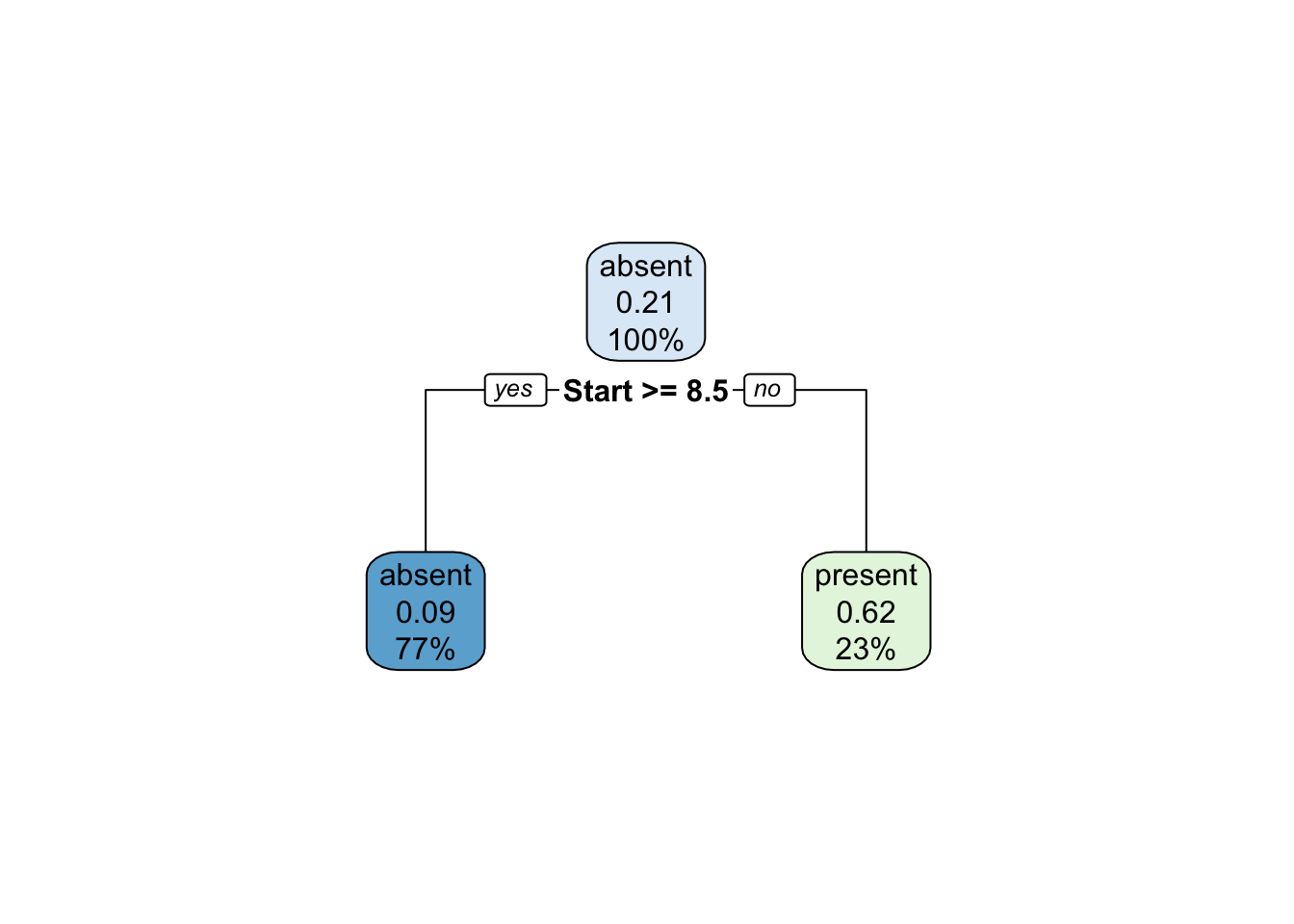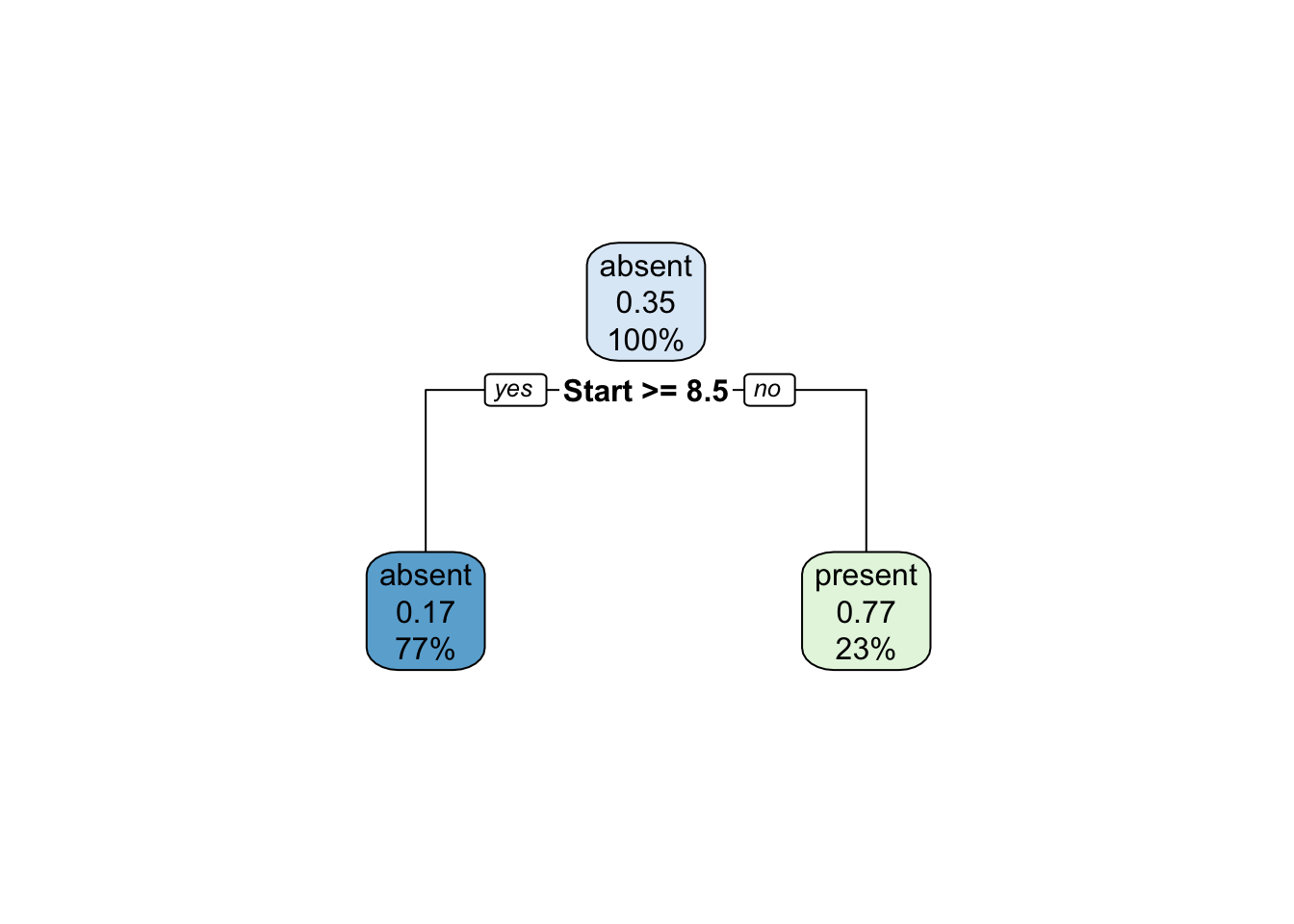# Important Dates/Information

Due date: Friday, March 2nd, 2018 11:59PM . No late submission allowed! Please submit to blackboard.

Update to submission guidelines: Submit both RMD and PDF files. R script files are not necessary, nor will they be accepted. Please submit all files in such format: _lab#_mmdd.extension ********

# Introduction

### Learning Goals

1. Intro to CART
2. Cross-validation in tree-based models
3. RandomForest

# Classification and Regression Trees

Decision trees are created by recursively partitioning the input space to categorize (or regress) data. Rules are generated for dividing data during training, and when used to test the rules are applied to the data and the according result applied as a classification or regression.

These bespoke conditions do an excellent job fitting a tree to a given training set of data. We would refer to this as a low bias learner. However we also know that with low bias comes high variance, and intuitively we can think that these decision rules may be rather arbitrary when applied to another set of data generated in the same way. In a regression example, imagine I had the following data and wayt to predict weight as a function of height and shirt with a tree.

weight <- c(150,165,167,190,225)
height <- c(4.5,5.5,5.6,6.1,7.0)
shirt  <- c("S","M","M","L","XL")

We can intuit rules about how to make a series of binary decisions to map height and shirt size to weight. Ie, a shirt size of S indicates weight is 150, a shirt size of M and a height less than or equal to 5.5 indicates weight 165. Shirt size of XL predicts weight of 225 etc…

This tree works perfectly to ‘memorize’ the training data, however would not necessarily perform well if we measured another 5 people. In R, we will use the rpart package to build single-tree model using our old friend the kyphosis data.

We are going to build a tree-based classification model for the prediction of Kyphosis. Let’s first load the rpart package. I then want to develop a training and test sample, and finally build the model. Below is one way to do that in R. Note we can specify information about the way the splitting is done by specifying prior information. In this case, we are splitting the data into two sections at the root node.

library('rpart')
library('rpart.plot')
data("kyphosis") # loda kyphosis data from rpart package
folds <-5

# add a column sampled from 1 to 5 which will be our fold
set.seed(1)
kyphosis$fold <- sample(x=1:folds,size=nrow(kyphosis),replace=T) # assign folds head(kyphosis) ## Kyphosis Age Number Start fold ## 1 absent 71 3 5 2 ## 2 absent 158 3 14 2 ## 3 present 128 4 5 3 ## 4 absent 2 5 1 5 ## 5 absent 1 4 15 2 ## 6 absent 1 2 16 5 # now let rows with fold==1 be our test set, the rest training kyphosis.train <- kyphosis[-which(kyphosis$fold==1),]
kyphosis.test  <- kyphosis[which(kyphosis$fold==1),] # build the model on the training set model1 <- rpart(Kyphosis~Age+Number+Start,data=kyphosis.train,method = "class") summary(model1) ## Call: ## rpart(formula = Kyphosis ~ Age + Number + Start, data = kyphosis.train, ## method = "class") ## n= 71 ## ## CP nsplit rel error xerror xstd ## 1 0.2666667 0 1.0000000 1.0000000 0.2293080 ## 2 0.0100000 1 0.7333333 0.7333333 0.2032598 ## ## Variable importance ## Start Number ## 84 16 ## ## Node number 1: 71 observations, complexity param=0.2666667 ## predicted class=absent expected loss=0.2112676 P(node) =1 ## class counts: 56 15 ## probabilities: 0.789 0.211 ## left son=2 (55 obs) right son=3 (16 obs) ## Primary splits: ## Start < 8.5 to the right, improve=7.071063, (0 missing) ## Age < 72 to the left, improve=2.172541, (0 missing) ## Number < 6.5 to the left, improve=2.014650, (0 missing) ## Surrogate splits: ## Number < 6.5 to the left, agree=0.817, adj=0.188, (0 split) ## ## Node number 2: 55 observations ## predicted class=absent expected loss=0.09090909 P(node) =0.7746479 ## class counts: 50 5 ## probabilities: 0.909 0.091 ## ## Node number 3: 16 observations ## predicted class=present expected loss=0.375 P(node) =0.2253521 ## class counts: 6 10 ## probabilities: 0.375 0.625 rpart.plot(model1)# now build specifying the root node split model2 <- rpart(Kyphosis ~ Age + Number + Start, data = kyphosis.train, parms = list(prior = c(.65,.35), split = "information")) summary(model2) ## Call: ## rpart(formula = Kyphosis ~ Age + Number + Start, data = kyphosis.train, ## parms = list(prior = c(0.65, 0.35), split = "information")) ## n= 71 ## ## CP nsplit rel error xerror xstd ## 1 0.4676871 0 1.0000000 1.0000000 0.2293080 ## 2 0.0100000 1 0.5323129 0.6653061 0.1709703 ## ## Variable importance ## Start Number ## 84 16 ## ## Node number 1: 71 observations, complexity param=0.4676871 ## predicted class=absent expected loss=0.35 P(node) =1 ## class counts: 56 15 ## probabilities: 0.650 0.350 ## left son=2 (55 obs) right son=3 (16 obs) ## Primary splits: ## Start < 8.5 to the right, improve=12.017470, (0 missing) ## Age < 39.5 to the left, improve= 5.436192, (0 missing) ## Number < 2.5 to the left, improve= 4.255204, (0 missing) ## Surrogate splits: ## Number < 6.5 to the left, agree=0.817, adj=0.188, (0 split) ## ## Node number 2: 55 observations ## predicted class=absent expected loss=0.1673783 P(node) =0.6970238 ## class counts: 50 5 ## probabilities: 0.833 0.167 ## ## Node number 3: 16 observations ## predicted class=present expected loss=0.2298625 P(node) =0.3029762 ## class counts: 6 10 ## probabilities: 0.230 0.770 rpart.plot(model2)The trouble with these trees -however- is their performance on out of sample data. We use the testing set developed above as our guide. Without loss of generality we will show the results of the tree on the training and test set. model1.pred.IS <- predict(model1,type="class") table(model1.pred.IS,kyphosis.train$Kyphosis)
##
## model1.pred.IS absent present
##        absent      50       5
##        present      6      10
model.1.pred.OS <- predict(model1,newdata=kyphosis.test,type="class")
table(model.1.pred.OS,kyphosis.test$Kyphosis) ## ## model.1.pred.OS absent present ## absent 6 1 ## present 2 1 In this instance the out of sample performance is not significantly worse but that’s due to our one-level trees. When performing this data with a larger sample space (or just plain more rows) we end up with more rules which creates less bias, but also more variance. To use trees effectively, a trimming procedure (similar to step.gam) should be applied to keep the number of variables and decisions to a minimum. # Random Forest One alternative to the previous tree on the data, would be to randomly sample the data and build trees on the larger aggregated set. When we use what we have to build more data, it is called bootstrapping samples. However, we still end up with only one tree to do the out of sample prediction so we are still prone to overfitting because we grow a tree ‘too deep.’ A clever way around this is to develop a series of trees and let their outputs “vote” on the correct answer. The natural name for a series of trees is a prediction forest. However as in the situation where we had one tree, we are still prone to overfitting due to a series of deep trees. The way around this is to only build the tree on a subset of variables. We generally choose some subset of variables (much less than the total number of variables) and predict only using those. In doing so, we can build the trees as large as we want but we still aren’t overfitting on the entire data. This is what puts the random in random forest. The idea is this 1. Sample with replacement from the training data 2. Randomly sample a small subset of varibles 3. Build a tree on the small subset of variables as large as possible Once that has been done a sufficiently large number of times, let the trees vote on the overall prediction. There are numerous benefits over other methods as to why random forest is a good call. I’ll leave it up to the experts to the selling. But in R the implementation is quite simple library('randomForest') ## randomForest 4.6-12 ## Type rfNews() to see new features/changes/bug fixes. model3 <- randomForest(Kyphosis~Age + Number+ Start, data=kyphosis.train) model3.pred.IS <- predict(model3,newdata=kyphosis.train) table(model3.pred.IS,kyphosis.train$Kyphosis)
##
## model3.pred.IS absent present
##        absent      56       0
##        present      0      15
model3.pred.OS <- predict(model3,newdata = kyphosis.test)
table(model3.pred.OS,kyphosis.test\$Kyphosis)
##
## model3.pred.OS absent present
##        absent       7       2
##        present      1       0

We can see immediatly that the in-sample performance is almost perfect with no out-of sample penalty versus a given tree. Personally, randomforest is one of my go-to prediction tools and can be generally counted on to do a good job. A caviat however, while the model does use ‘out of bag’ error estimates we still need to cross validate as a measure of good practice. This is because no amount of bagging will generate outliers (remember Los Alamos County??) so we have to use and evaluate everything we have.

# Questions

NOTE: To further emphasize the importance of visual presentation, 1/3 of the lab grades from now on will be based on presentation. This includes things like the quality of the arguments used, the completeness of text-based answers, and the overall feel of the submitted document.

For these questions, we will be using the TCCount dataset from blackboard. This is taken from Sabbatelli and Mann 2007 (DOI: 10.1029/2007JD008385, an interesting read about how climate scientists use statistics). The column headers are “Year”, “TS” which is the number of tropical storms, “LF.count” which is the number of landfalling tropiclal storms, “SST” or Sea Surface Temperature, “ENSO” the EL Nino Index, and finally “NAO” or the North Atlantic Oscillator index.

All of these have some physical motivation behind why they might increase the number of tropcal storms during a given season.

1. Using only one 80/20% training/test split of the data, build a tree using rpart to fit the number of Tropical Storms using SST, ENSO and NAO. How does the model perform in sample and out of sample? Include any plots you may find helpful. (2 points)

2. Now add a column to the TC dataset which is 1 if the number of tropical storms is greater than the 80th percentile value of the data and 0 otherwise. Perform a 10-fold cross validation in which you build a model to predict whether this new variable is a 1 or 0 using NAO, SST and ENSO. Show the percentage of correct predictions for each cross validation and discuss whether the results of question 1 hold. (2 points)

3. Build a randomForest model to predict tropical storms with ENSO, SST, and NAO. Perform the same cross validation as in question 2 but this time do both rpart and randomForest. Based on these cross validated results, discuss which model is better and why. (3 points)

The remaining 3 points will be awarded based on your discussion, and how well you present what you have done. Receiving all 3 of these points will be awarded if you can clearly and persuasively communicate your ideas. Completeing the assignment as it is written and just providing answers might get you a 1.